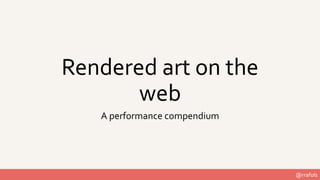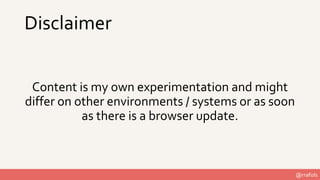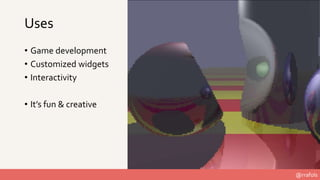Successfully reported this slideshow.

# Rendering Art on the Web - A Performance compendium×

1 of 61 Ad
1 of 61 Ad

# Rendering Art on the Web - A Performance compendium

This session starts by showing how to build something very simple with the HTML5 Canvas API, taking into account some performance considerations. Then the presentation adds more rendering complexity while keeping an eye on how it is impacting performance. It also evaluates different alternatives from a performance point of view and tries out a few optimizations. Having the right level of optimization makes it possible to add more complexity or render what seemed impossible. On the other hand, not optimizing properly will definitely impact performance and reduce quality, and for mobile users, battery life will suffer more than it needs to. As somebody said before, “With great power comes great responsibility.”

This session starts by showing how to build something very simple with the HTML5 Canvas API, taking into account some performance considerations. Then the presentation adds more rendering complexity while keeping an eye on how it is impacting performance. It also evaluates different alternatives from a performance point of view and tries out a few optimizations. Having the right level of optimization makes it possible to add more complexity or render what seemed impossible. On the other hand, not optimizing properly will definitely impact performance and reduce quality, and for mobile users, battery life will suffer more than it needs to. As somebody said before, “With great power comes great responsibility.”

### Rendering Art on the Web - A Performance compendium

1. 1. @rrafols Rendered art on the web A performance compendium
2. 2. @rrafols Disclaimer Content is my own experimentation and might differ on other environments / systems or as soon as there is a browser update.
3. 3. @rrafols Uses • Game development • Customized widgets • Interactivity • It’s fun & creative
4. 4. @rrafols • JS1k - https://js1k.com/ • Demoscene - https://en.wikipedia.org/wiki/Demoscene • P01 - http://www.p01.org/about/ • JS13k games - https://js13kgames.com/ • Creative JS - http://creativejs.com/
5. 5. @rrafols Let’s build something simple to begin with……
6. 6. @rrafols Step 0 - Recursive tree • Set up a <canvas> • Initialize the rendering loop with requestAnimationFrame()
7. 7. @rrafols Step 0 - Recursive tree let a = document.getElementsByTagName('canvas') let c = a.getContext('2d') let w = window.innerWidth let h = window.innerHeight render = ts => { requestAnimationFrame(render) } requestAnimationFrame(render)
8. 8. @rrafols Step 1 - Recursive tree • Clear the <canvas> on each iteration • Draw the initial line
9. 9. @rrafols Step 1 - Recursive tree a.width = w a.height = h c.strokeStyle = '#fff' c.beginPath() c.moveTo(w/2, h) c.lineTo(w/2, h - h/3) c.stroke()
10. 10. @rrafols Step 2 - Recursive tree • Convert the drawing into a parametrized function • Lines direction modified by an angle
11. 11. @rrafols Step 2 - Recursive tree split(w / 2, h, h / 3, Math.PI / 2) split = (x, y, length, angle) => { let x1 = x + length * Math.cos(angle) let y1 = y - length * Math.sin(angle) c.beginPath() c.moveTo(x, y) c.lineTo(x1, y1) c.stroke() }
12. 12. @rrafols Step 3 - Recursive tree • Call the split function recursively • Modify the angle in each iteration to build the tree structure
13. 13. @rrafols Step 3 - Recursive tree c.beginPath() c.moveTo(x, y) c.lineTo(x1, y1) c.stroke() split(x1, y1, length/1.5, angle - Math.PI/4, it + 1) split(x1, y1, length/1.5, angle + Math.PI/4, it + 1)
14. 14. @rrafols Step 4 - Recursive tree • Add more branches / bifurcations • Increase max number of iterations • Count the number of lines drawn so we can estimate drawing complexity
15. 15. @rrafols Step 4 - Recursive tree lines++ … split(x1, y1, length/1.5, angle - Math.PI/3, it + 1) split(x1, y1, length/1.5, angle - Math.PI/8, it + 1) split(x1, y1, length/1.5, angle + Math.PI/3, it + 1) split(x1, y1, length/1.5, angle + Math.PI/8, it + 1) … c.fillStyle = '#fff' c.fillText("lines: " + lines, w - 200, 20)
16. 16. @rrafols Step 5 - Recursive tree • Animate tree movement • Recursively displace branches for intensified effect • Apply alpha for smoother effect
17. 17. @rrafols Step 5 - Recursive tree c.globalAlpha = 0.2 c.strokeStyle = '#fff' let angle = Math.PI * Math.sin(ts * 0.0003)*0.15 + Math.PI/2 split(w/2, h, h/3, angle, Math.cos(ts * 0.0004) * Math.PI/8, 0) c.globalAlpha = 1
18. 18. @rrafols Step 6 - Recursive tree • Increase maximum number of iterations and see what happens…
19. 19. @rrafols <code>
20. 20. @rrafols How can we measure performance? Feels slower right? To measure the frames per second we can do any of the following: • Implement a simple frame counter • Web Developer tools in Chrome & Firefox • Available open source libraries such as stats.js • https://github.com/mrdoob/stats.js/ • Use jsPerf to create test & run cases • https://jsperf.com/
21. 21. @rrafols
22. 22. @rrafols
23. 23. @rrafols Using stats.js 1) Use npm to install it: rrafols\$ npm install stats.js 2) Include into the source file <script src="node_modules/stats.js/build/stats.min.js"/> 3) Add it to the render function:
24. 24. @rrafols Adding stats.js var stats = new Stats() stats.showPanel(0) document.body.appendChild( stats.dom ) … render = ts => { stats.begin() … stats.end() requestAnimationFrame(render) }
25. 25. @rrafols Step 7 – Recursive tree Now that we know it is slower, what we can do about it? How can we optimize it? • …
26. 26. @rrafols Step 7 – Recursive tree Now that we know it is slower, what we can do about it? How can we optimize it? • Draw one single path with all the lines in it instead of several paths with one single line. • …
27. 27. @rrafols Step 7 - Recursive tree // c.beginPath() c.moveTo(x, y) c.lineTo(x1, y1) // c.stroke() … c.beginPath() let angle = Math.PI*Math.sin(ts * 0.0003)*0.15… split(w/2, h, h/3, angle, Math.cos(ts * 0.0004)… c.stroke()
28. 28. @rrafols Step 7 – Recursive tree Now that we know it is slower, what we can do about it? How can we optimize it? • Draw one single path with all the lines in it instead of several paths with one single line. • Let’s increase the number of iterations to see how it behaves now • …
29. 29. @rrafols <demo>
30. 30. @rrafols Performance Profiling
31. 31. @rrafols Performance Profiling There might be a CPU bottleneck when calling recursively the split function. Let’s check if that is the issue…
32. 32. @rrafols Performance profiling let path = new Path2D() … path.moveTo(x, y) path.lineTo(x1, y1) … c.strokeStyle = '#fff' c.stroke(path)
33. 33. @rrafols Performance Profiling There is an impact in the frame rate by function calling overhead, but rendering seems to be the bottleneck
34. 34. @rrafols Let’s try something else
35. 35. @rrafols Grid We have several ways of drawing a grid, let’s see some of them: • Using strokeRect directly on the context • Adding rect to a path and stroking that path • Generating a path with moveTo / lineTo instead of rect • ...
36. 36. @rrafols Grid - strokeRect for(let i = 0; i < h / GRID_SIZE; i++) { for(let j = 0; j < w / GRID_SIZE; j++) { let x = j * GRID_SIZE let y = i * GRID_SIZE c.strokeRect(x, y, GRID_SIZE, GRID_SIZE) } }
37. 37. @rrafols Grid – path & rect let path = new Path2D() … for(let i = 0; i < h / GRID_SIZE; i++) { for(let j = 0; j < w / GRID_SIZE; j++) { let x = j * GRID_SIZE let y = i * GRID_SIZE path.rect(x, y, GRID_SIZE, GRID_SIZE) } } … c.stroke(path)
38. 38. @rrafols Grid – moveTo/lineTo c.beginPath() for(let i = 0; i < h / GRID_SIZE; i++) { for(let j = 0; j < w / GRID_SIZE; j++) { let x = j * GRID_SIZE let y = i * GRID_SIZE c.moveTo(x, y) c.lineTo(x + GRID_SIZE, y) c.lineTo(x + GRID_SIZE, y + GRID_SIZE) c.lineTo(x, y + GRID_SIZE) c.lineTo(x, y) } } c.stroke()
39. 39. @rrafols Grid – moveTo/lineTo - path let path = new Path2D() for(let i = 0; i < h / GRID_SIZE; i++) { for(let j = 0; j < w / GRID_SIZE; j++) { let x = j * GRID_SIZE let y = i * GRID_SIZE path.moveTo(x, y) path.lineTo(x + GRID_SIZE, y) path.lineTo(x + GRID_SIZE, y + GRID_SIZE) path.lineTo(x, y + GRID_SIZE) path.lineTo(x, y) } } c.stroke(path)
40. 40. @rrafols <demo>
41. 41. @rrafols Grid – transformation c.save() c.translate(w / 2, h / 2) c.rotate(angle) c.translate(-w / 2, -h / 2) … c.restore()
42. 42. @rrafols Grid – transformation //c.save() c.translate(w / 2, h / 2) c.rotate(angle) c.translate(-w / 2, -h / 2) … //c.restore() c.setTransform(1, 0, 0, 1, 0, 0)
43. 43. @rrafols <code>
44. 44. @rrafols Grid
45. 45. @rrafols Grid – transformation rotate = (x, y, angle) => { x -= w/2 y -= h/2 return [ x * Math.cos(angle) - y * Math.sin(angle) + w/2, y * Math.cos(angle) + x * Math.sin(angle) + h/2 ] }
46. 46. @rrafols <demo>
47. 47. @rrafols OKNOK strokeRect path.rect path.lineT o c.lineTo
48. 48. @rrafols Grid What about fill operations instead of stroke? Let’s fill the rects to see the differences.
49. 49. @rrafols <demo>
50. 50. @rrafols OK NOK fillRect path.rect path.lineT o c.lineTo
51. 51. @rrafols Grid What about images? ImageAtlas vs single images Images: https://toen.itch.io/toens-medieval-strategy
52. 52. @rrafols ImageAtlas – drawing & clipping c.save() c.beginPath() c.rect(j * GRID_SIZE, i * GRID_SIZE, GRID_SIZE, GRID_SIZE) c.clip() c.drawImage(image, j * GRID_SIZE - 64, i * GRID_SIZE)
53. 53. @rrafols <demo>
54. 54. @rrafols Grid – ImageAtlas vs single images
55. 55. @rrafols Grid – image smoothing Browsers smooth images when drawing on decimal positions: c.drawImage(image, 5.24, 10.23)
56. 56. @rrafols ImageAtlas – drawing on exact pixels c.drawImage(imageLarge, (w / 2 - imageLarge.naturalWidth / 2) |0, (h / 2 - imageLarge.naturalHeight / 2) |0)
57. 57. @rrafols <demo>
58. 58. @rrafols Images: https://dragosha.com/free/adventure-tileset.html
59. 59. @rrafols Conclusions • Avoid allocating memory inside render loop – GC is “evil”! • Group paths together rather than drawing multiple small paths • Pre-calculate & store as much as possible • Values & numbers • Paths, Gradients, … • Reduce render state machine changes • Careful with canvas transformations • Reset transformation with setTransform instead of save/restore. • Always measure & profile. Check differences on several browsers.
60. 60. @rrafols Thank you • More information: https://developer.mozilla.org/en-US/docs/Web/API/Canvas_API/Tutorial • Source code: https://github.com/rrafols/html_canvas_performance • Contact: https://www.linkedin.com/in/raimonrafols/
61. 61. @rrafols <?>